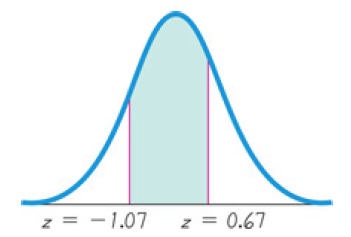×
Get Full Access to Elementary Statistics - 12 Edition - Chapter 6.2 - Problem 12bsc
Get Full Access to Elementary Statistics - 12 Edition - Chapter 6.2 - Problem 12bsc

×

# Solution: Standard Normal Distribution. In Exercises, findISBN: 9780321836960 18

## Solution for problem 12BSC Chapter 6.2

Elementary Statistics | 12th Edition

• Textbook Solutions
• 2901 Step-by-step solutions solved by professors and subject experts
• Get 24/7 help from StudySoup virtual teaching assistantsElementary Statistics | 12th Edition

4 5 1 314 Reviews
11
3
Problem 12BSC

Standard Normal Distribution. In Exercises 9–12, find the area of the shaded region. The graph depicts the standard normal distribution of bone density scores with mean 0 and standard deviation 1.Step-by-Step Solution:
Step 1 of 3

Problem 12BSC

Step1 of 2:

We have In Exercises, find the area of the shaded region. The graph depicts the standard normal distribution of bone density scores with mean 0 and standard deviation 1.Step2 of 2:

From the above graph we have P(p < z <q ).

P(p < z <q ) = P(-1.07 < z < 0.67)

= P(z < q) - P(z < p)

= P(z < 0.67) - P(z < -1.07)

area under normal curve can be calculated by using standard normal table or statistical table that is P(z < 0.67) = 0.7486 (i.e, row 0.6 under column 0.07)

P(z < -1.07) = 0.1423 (i.e, row -1.0 under column 0.07)

consider,

P(-1.07 < z < 0.67) = P(z < 0.67) - P(z < -1.07)

= 0.7486 - 0.1423

= 0.6063.

Therefore, the area of the shaded region is 0.6063.

Step 2 of 3

Step 3 of 3

##### ISBN: 9780321836960

Unlock Textbook Solution